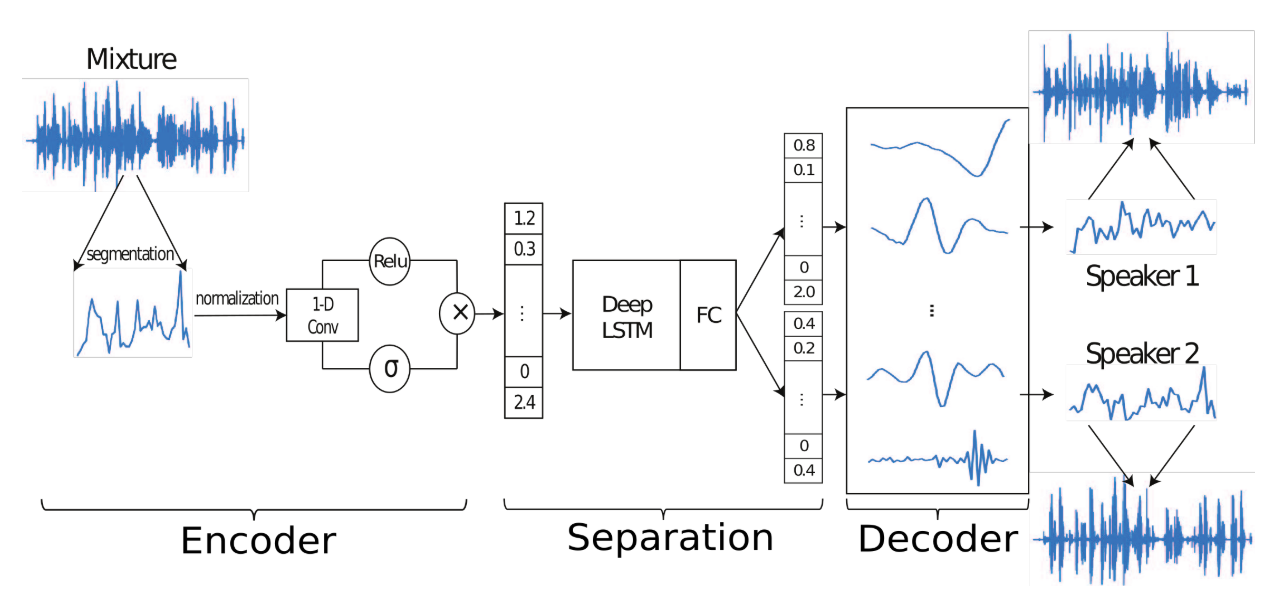# Latent Alignment and Variational Attention

## WHY?

Even though attention is being widely used, it is hard to be considered as probabilistic model as the attention does not marginalize.

## WHAT?

This paper formulated source separation task as getting mixture meight vector of multiple sources in wave form.

x(t) = \sum_{i=1}^C s_i(t)\\
x = wB\\
s_i = d_iB\\
w = \sum_{i=1}^C d_i = \sum_{i=1}^C w \odot (d_i \oslash w) := w \odot \sum_{i=1}^C m_i\\
d_i = m_i \odot w

Time-domain Audio Separation Network(TasNet) tries to find m_i$m_i$ which is relative contribution to each w while B is N basis signals of shape N x L.Encoder find w for B by appling 1-D gated convolution layer.

w_k = ReLU(x_k \circledast U)\odot\sigma(x_k\circledast V)\\

Separation network uses LSTM and FC for masks(m_i$m_i$) generation. With w and m found above, d can be found with decoder. The scale-invariant source-to-noise ratio(SI-SNR) is used for loss.

## So?TasNet not only showed comparable performance in WSJ0-2mix dataset, but also showen to find its own basis.

Powered by aiden